# Model obesity levels using machine learning

This example aims to assess obesity levels in individuals from the countries of Mexico, Peru and Colombia, based on their eating habits and physical condition in order to give a treatment to the patient.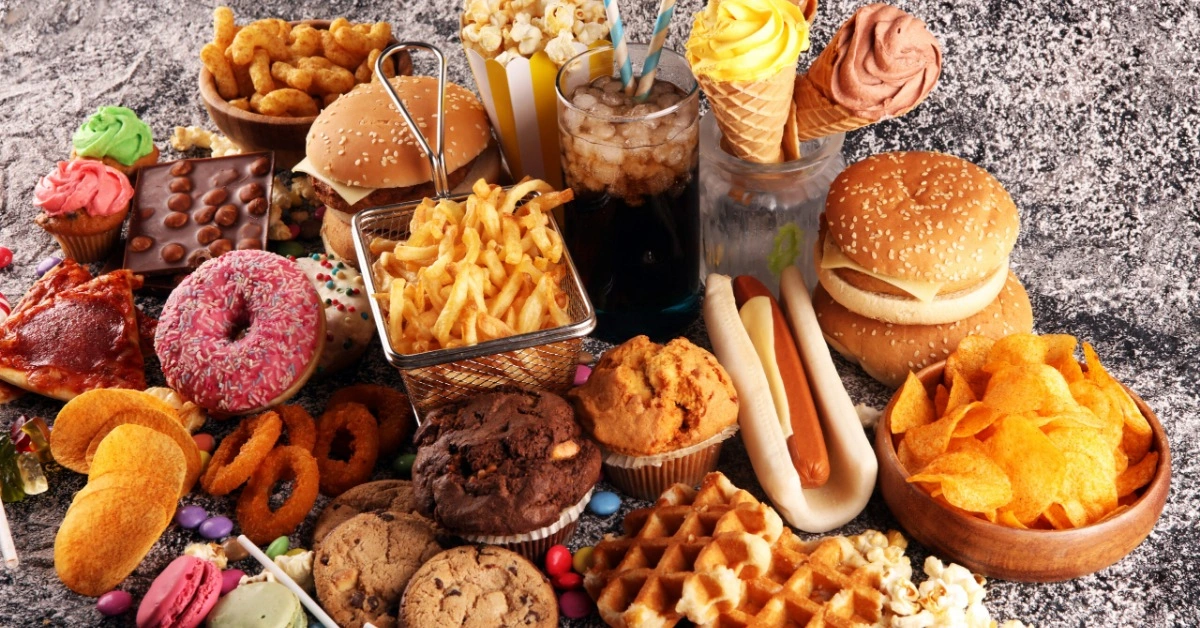### Contents:

This example is solved with Neural Designer. To follow it step by step, you can use the free trial.

## 1. Application type

The variable to be predicted is continuous (Insufficient Weight, Normal Weight, Overweight Level I, Overweight Level II, Obesity Type I, Obesity Type II and Obesity Type III). Therefore, this is an approximation project.

Here, the basic goal is to model the obesity levels as a function of the input variables and advised tha patient what to do in order to improve obesity level.

## 2. Data set

The data set contains three concepts:

• Data source.
• Variables.
• Instances.

The ObesityDataSet.csv file contains the data for this application. The number of instances (rows) in the data set is 2111, and the number of variables (columns) is 17.

The number of input variables, or attributes for each sample, is 14. Height and weight are unused variables because they are totally related with the target variable. The input variables are numeric-valued, binary and categorical. The number of target variables is 1 and represents the estimation of obesity levels in individuals. The following list summarizes the variables information:

• gender: (1=Female or 0=Male).
• age: (Numeric).
• height: (Numeric).
• weight: (Numeric).
• family_history_with_overwight: (1=Yes/0=No).
• caloric_food:(0=Yes/1=No). Frequent consumption of high caloric food.
• vegatables: (1, 2 or 3). Frequency of consumption of vegetables.
• number_meals: (1, 2, 3 or 4). Number of main meals.
• food_between_meals: (1=No, 2=Sometimes, 3=Frequently or 4=Always). Consumption of food between meals.
• smoke: (0=Yes/1=No).
• water: (1, 2 or 3). Consumption of water daily.
• calories: (0=Yes/1=No).Calories consumption monitoring.
• activity: (0, 1, 2 or 3). Physical activity frequency.
• technology: (0, 1 or 2). Time using technology devices.
• alcohol: (1=No, 2=Sometimes, 3=Frequently or 4=Always). Consumption of alcohol.
• transportation: (Automobile, motorbike, bike, public transportation or walking). Transportation used.
• obesity_level: (1=Insufficient_Weight, 2=Normal_Weight, 3=Overweight_Level_I, 4=Overweight_Level_II, 5=Obesity_Type_I, 6=Obesity_Type_II, 7=Obesity_Type_III)

Finally, the use of all instances is set. Note that each instance contains the input and target variables of a different patient. The data set is divided into training, validation, and testing subsets. 60% of the instances will be assigned for training, 20% for generalization, and 20% for testing. More specifically, 1267 samples are used here for training, 422 for selection and 422 for testing samples.

Once the data set has been set, we are ready to perform a few related analytics. With that, we check the provided information and make sure that the data has good quality.

We can calculate the distributions of the variables. The following chart shows the histogram for the obesity level.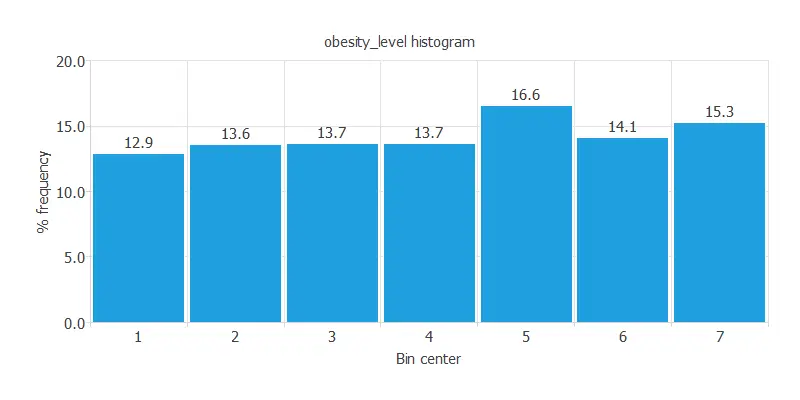As we can see, the obesity level has a semi-normal distribution. The maximum frequency is 16.6272%, which corresponds to the bin with center 5. The minimum frequency is 12.8849%, which corresponds to the bin with center 1.

The inputs-targets correlations might indicate to us what factors most influence patients' obesity level.Here, the most correlated variables with obesity levels are caloric food, family_history_with_overweight and age.

## 3. Neural network

The third step is to set the model parameters. For approximation project type, it is composed of:

• Scaling layer.
• Perceptron layers.
• Unscaling layer.

The mean and standard deviation is set as the scaling method, while the minimum and maximum is set as the unscaling method. The activation function chosen for this model is the hyperbolic tangent activation function and the linear activation function for the hidden layer and the output layer, respectively.

A graphical representation of the neural network is depicted next.It contains a scaling layer, 2 perceptron layers, and an unscaling layer. The number of inputs is 18, and the number of outputs is 1. The complexity, represented by the numbers of neurons in the hidden layer is 3.

## 4. Training strategy

The fourth step is to set the training strategy, which is composed of two terms:

• A loss index.
• An optimization algorithm.

The learning problem can be stated as finding a neural network that minimizes the loss index. That is, a neural network that fits the data set (error term) and does not oscillate (regularization term).

The loss index is the normalized squared error with L1 regularization.

The optimization algorithm that we use is the quasi-Newton method. This is also the standard optimization algorithm for this type of problem.This chart shows how the error decreases with the iterations during the training process. The final training and selection errors are training error = 0.0176 WSE and selection error = 0.0236 WSE, respectively.

## 5. Model selection

The objective of model selection is to improve the generalization capabilities of the neural network or, in other words, to reduce the selection error.

Since the selection error that we have achieved so far is very small (0.0236 NSE), we don't need to apply order selection nor input selection here.

## 6. Testing analysis

Once the model is trained, we perform a testing analysis to validate its prediction capacity. This will be done by comparing the neural network outputs against the real target values for a set of data never seen before. The testing analysis will determine if the model is ready to move to the production phase.

The next chart illustrates the linear regression analysis for the variable particles_adhering.For a perfect fit, the values of the intercept, slope, and correlation should be 0, 1, and 1. In this case, we have intercept = 0.263, slope = 0.947 and correlation = 0.844. The achieved values are close to the ideal ones, so the model shows a good performance.

## 7. Model deployment

Once the neural network's generalization performance has been tested, the neural network can be saved for future use in the so-called model deployment mode.

We can treat new patients by calculating the neural network outputs. For that we need to know patients next details. Here we have a new patient:

• gender: Male.
• age: 35.
• family_history_with_overwight: Yes.
• caloric_food: Yes.
• vegatables: 2.
• number_meals: 2.
• food_between_meals: No.
• smoke: Yes.
• water: 2.
• calories: Yes.
• activity: 1.
• technology: 1.
• alcohol: Frequently.
• transportation: public_transportation.
• obesity_level: 5.406 = Obesity_Type_I.

We can plot directional outputs to study the behavior of the output variable obesity_level as the function of single inputs.

As we see in calculating correlations, the inputs that most influence obesity_level are weight and height. If patients' height increases obesity level drecreases and the inverse happenes with the weight.

Despite those two attributes, this patient can reduce obesity level by changing two of her habits. As the obesity level is not a problem that can be solved from one day to another, the treatment must be applied little by little. The treatment will be to increase the number of food between meals and have more activity. We can see it below: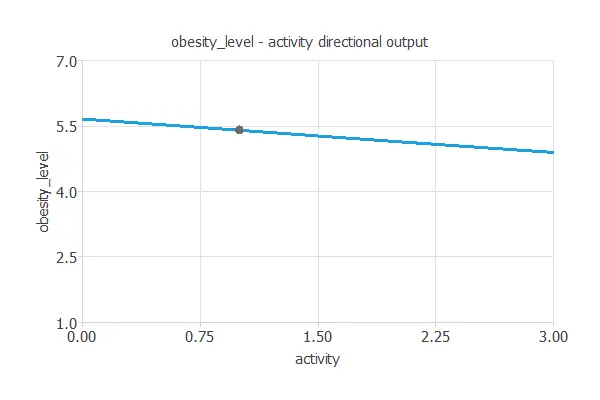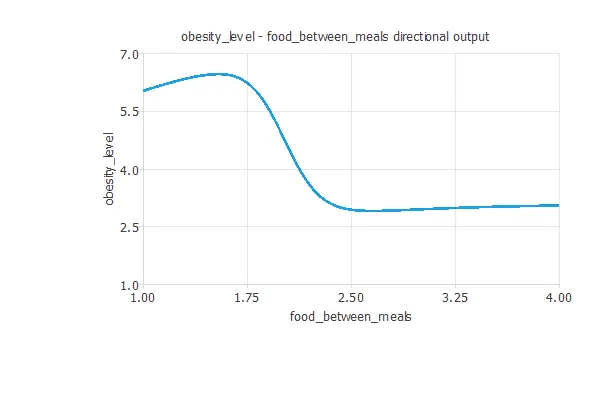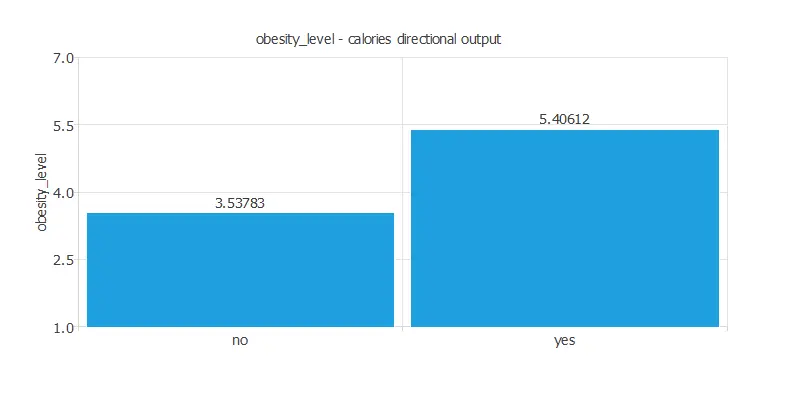The first plot shows the output obesity level as a function of the input activity. The second one represents the output obesity level as a function of the input food between meals. The last graph represents that if the patient consumes more calories, obesity level is higher.

To sum up, the treatment for this patient is:

• increase the number of food between meals
• more activity such as walking at least 30mins each day
• decrease calories consumption monitoring

Besides, we can use the mathematical expression of the neural network, which is listed next.

```scaled_gender = (gender-(0.4940789938))/0.5000830293;
scaled_age = age*(1+1)/(61-(14))-14*(1+1)/(61-14)-1;
scaled_family_history_with_overweight = family_history_with_overweight*(1+1)/(1-(0))-0*(1+1)/(1-0)-1;
scaled_caloric_food = (caloric_food-(0.1160589978))/0.320371002;
scaled_vegetables = (vegetables-(2.419039965))/0.5339270234;
scaled_number_meals = number_meals*(1+1)/(4-(1))-1*(1+1)/(4-1)-1;
scaled_food_between_meals = (food_between_meals-(2.140690088))/0.4685429931;
scaled_smoke = smoke*(1+1)/(1-(0))-0*(1+1)/(1-0)-1;
scaled_water = (water-(2.008009911))/0.6129530072;
scaled_calories = calories*(1+1)/(1-(0))-0*(1+1)/(1-0)-1;
scaled_activity = (activity-(1.01030004))/0.8505920172;
scaled_technology = (technology-(0.6578660011))/0.6089270115;
scaled_alcohol = (alcohol-(1.731410027))/0.5154979825;
scaled_public_transportation = (public_transportation-(0.7484599948))/0.4340009987;
scaled_walking = walking*(1+1)/(1-(0))-0*(1+1)/(1-0)-1;
scaled_automobile = (automobile-(0.2164849937))/0.4119459987;
scaled_motorbike = motorbike*(1+1)/(1-(0))-0*(1+1)/(1-0)-1;
scaled_bike = bike*(1+1)/(1-(0))-0*(1+1)/(1-0)-1;

perceptron_layer_0_output_0 = tanh[ -1.16742 + (scaled_gender*0.68282)+ (scaled_age*-0.420455)+ (scaled_family_history_with_overweight*0.691903)+ (scaled_caloric_food*-0.244554)+ (scaled_vegetables*0.769029)+ (scaled_number_meals*0.546811)+ (scaled_food_between_meals*-0.593264)+ (scaled_smoke*-0.250848)+ (scaled_water*-0.00675811)+ (scaled_calories*0.564604)+ (scaled_activity*0.0689401)+ (scaled_technology*0.0396549)+ (scaled_alcohol*-0.0964615)+ (scaled_public_transportation*0.839636)+ (scaled_walking*0.748955)+ (scaled_automobile*0.268782)+ (scaled_motorbike*0.528234)+ (scaled_bike*1.18717) ];
perceptron_layer_0_output_1 = tanh[ 0.32342 + (scaled_gender*-0.195494)+ (scaled_age*0.686579)+ (scaled_family_history_with_overweight*0.0506046)+ (scaled_caloric_food*0.00697568)+ (scaled_vegetables*-0.0795011)+ (scaled_number_meals*-0.0896854)+ (scaled_food_between_meals*0.294397)+ (scaled_smoke*-0.0825197)+ (scaled_water*-0.0133305)+ (scaled_calories*0.0261315)+ (scaled_activity*-0.0911458)+ (scaled_technology*0.010449)+ (scaled_alcohol*0.0786926)+ (scaled_public_transportation*-0.1329)+ (scaled_walking*-0.293047)+ (scaled_automobile*-0.286349)+ (scaled_motorbike*-0.128273)+ (scaled_bike*-0.160357) ];
perceptron_layer_0_output_2 = tanh[ -0.392613 + (scaled_gender*-0.367176)+ (scaled_age*-0.808962)+ (scaled_family_history_with_overweight*-0.384984)+ (scaled_caloric_food*0.131679)+ (scaled_vegetables*-0.0930502)+ (scaled_number_meals*0.221046)+ (scaled_food_between_meals*1.75847)+ (scaled_smoke*0.0215909)+ (scaled_water*-0.178107)+ (scaled_calories*0.513794)+ (scaled_activity*-0.360404)+ (scaled_technology*0.210205)+ (scaled_alcohol*0.451744)+ (scaled_public_transportation*0.289853)+ (scaled_walking*-0.0703812)+ (scaled_automobile*-0.570434)+ (scaled_motorbike*0.979235)+ (scaled_bike*0.448767) ];

perceptron_layer_1_output_0 = [ 0.179259 + (perceptron_layer_0_output_0*1.37047)+ (perceptron_layer_0_output_1*1.60426)+ (perceptron_layer_0_output_2*-0.714662) ];

unscaling_layer_output_0 = perceptron_layer_1_output_0*(7-1)/(1+1)+1+1*(7-1)/(1+1);

```

The file obesity.py implements the mathematical expression of the neural network in Python. This piece of software can be embedded in any tool to make predictions on new data.

## 8. Tutorial video

You can watch the step by step tutorial video below to help you complete this Machine Learning example for free using the easy-to-use machine learning software Neural Designer.

## References:

• The data for this problem has been taken from the UCI Machine Learning Repository.
• Palechor, F. M., & de la Hoz Manotas, A. (2019). Dataset for estimation of obesity levels based on eating habits and physical condition in individuals from Colombia, Peru and Mexico. Data in Brief, 104344.
• De-La-Hoz-Correa, E., Mendoza Palechor, F., De-La-Hoz-Manotas, A., Morales Ortega, R., & Sanchez Hernandez, A. B. (2019). Obesity level estimation software based on decision trees.